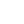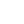Preview the Course
1h

# Graphing Lines and Parabolas

by Karissa Fong | Math FREE7 Lessons 1:00:48
1.1. Trailer 0:31
2.2. Introduction 0:27
3.3. Lines: How to Write the Equation of a Line and Graph It 27:07
4.4. Activity: Practice Problems 4:26
5.5. Parabolas: How to Write the Equation of a Parabola and Graph It 21:00
6.6. Activity: Practice Problems 6:15
7.7. Summary & Closing 1:02

1h

NGSS Standards

FREE

Course

Learn how to write and graph the equation of a line in Slope Intercept Form, Point Slope Form, and Standard Form. Use Vertex Form and Standard for to write and graph the equation of a parabola.

Tags:

• Equations
• Graph
• Lines
• Math
• Parabolas